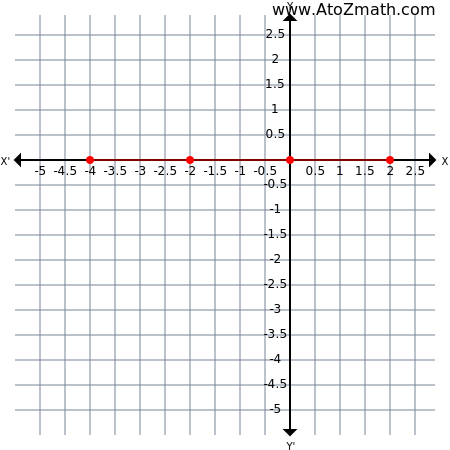Home > Geometry calculators > Coordinate Geometry > Points (3 or 4) are Collinear or Triangle or Quadrilateral form

 Solve any problem (step by step solutions) Input table (Matrix, Statistics)
Mode :
SolutionHelp
Solution
 Find collinear A(0,0),B(2,0),C(-4,0),D(-2,0) [ Calculator, Method and examples ]Solution:Your problem -> collinear A(0,0),B(2,0),C(-4,0),D(-2,0)Here A(0,0), B(2,0), C(-4,0), D(-2,0) are the given pointsCD^2 = (-2+4)^2 + (0-0)^2 = (2)^2 + (0)^2 = 4 + 0 = 4:. CD = sqrt(4) = 2DA^2 = (0+2)^2 + (0-0)^2 = (2)^2 + (0)^2 = 4 + 0 = 4:. DA = sqrt(4) = 2AB^2 = (2-0)^2 + (0-0)^2 = (2)^2 + (0)^2 = 4 + 0 = 4:. AB = sqrt(4) = 2CB^2 = (2+4)^2 + (0-0)^2 = (6)^2 + (0)^2 = 36 + 0 = 36:. CB = sqrt(36) = 6:. CD + DA + AB = 2+2+2=6=CB:. C,D,A,B are collinear pointsSolution provided by AtoZmath.com
Any wrong solution, solution improvement, feedback then Submit Here
Want to know about AtoZmath.com and me